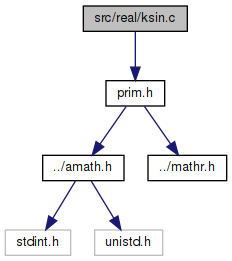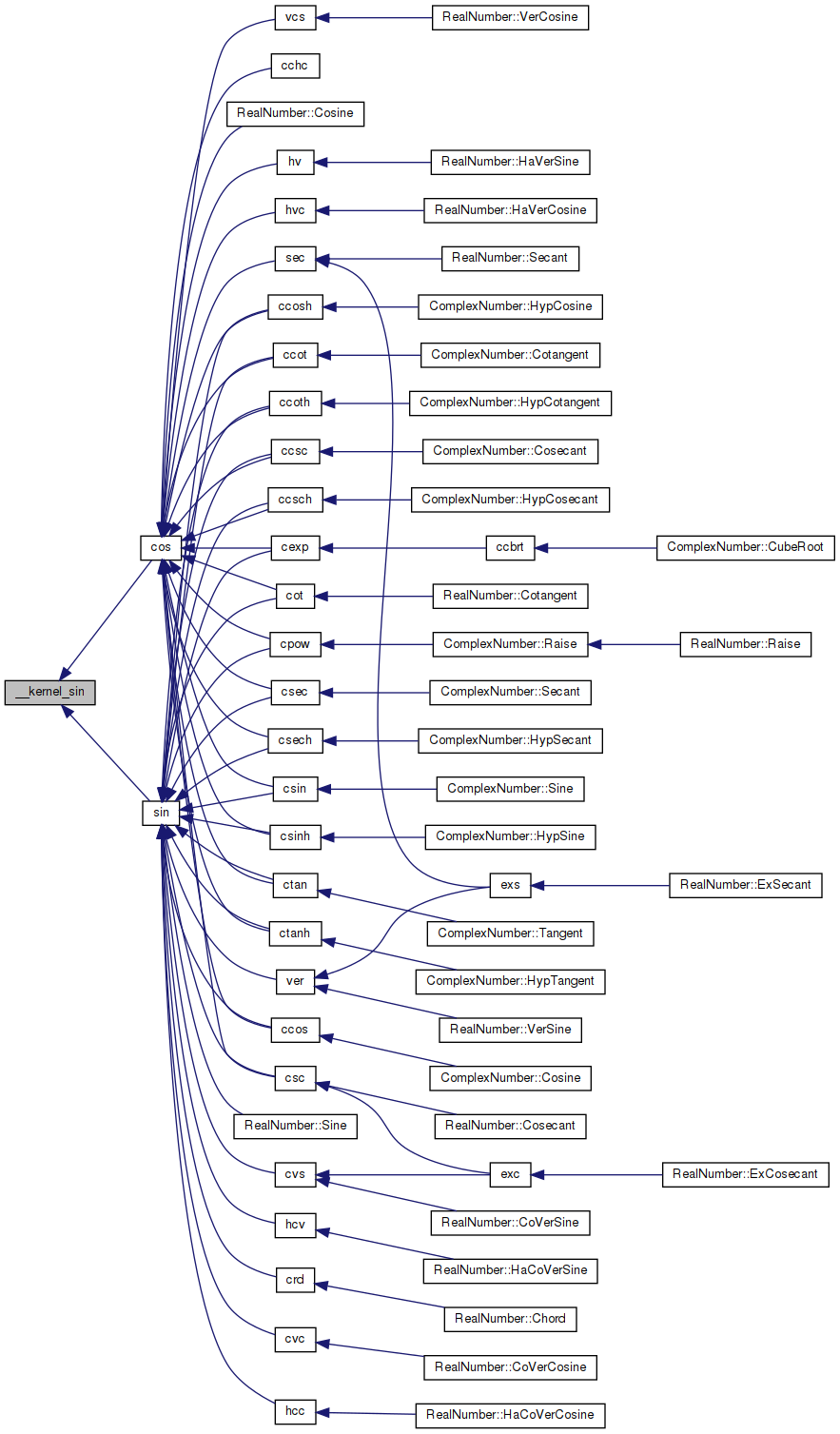amath  1.8.5 Simple command line calculator
ksin.c File Reference

Kernel sin function. More...

`#include "prim.h"`
Include dependency graph for ksin.c:Go to the source code of this file.

## Functions

double __kernel_sin (double x, double y, int iy)
Kernel sin function. More...

## Variables

static const double half = 5.00000000000000000000e-01

static const double S1 = -1.66666666666666324348e-01

static const double S2 = 8.33333333332248946124e-03

static const double S3 = -1.98412698298579493134e-04

static const double S4 = 2.75573137070700676789e-06

static const double S5 = -2.50507602534068634195e-08

static const double S6 = 1.58969099521155010221e-10

## Detailed Description

Kernel sin function.

Definition in file ksin.c.

## ◆ __kernel_sin()

 double __kernel_sin ( double x, double y, int iy )

Kernel sin function.

```Kernel sin function on [-pi/4, pi/4], pi/4 ~ 0.7854
Input x is assumed to be bounded by ~pi/4 in magnitude.
Input y is the tail of x.
Input iy indicates whether y is 0. (if iy=0, y assume to be 0).```
```Algorithm
1. Since sin(-x) = -sin(x), we need only to consider positive x.
2. if x < 2^-27 (hx<0X3E400000 0), return x with inexact if x!=0.
3. sin(x) is approximated by a polynomial of degree 13 on [0,pi/4]
3            13
sin(x) ~ x + S1*x + ... + S6*x```
`    where`
```    |sin(x)         2     4     6     8     10     12  |     -58
|----- - (1+S1*x +S2*x +S3*x +S4*x +S5*x  +S6*x   )| <= 2
|  x                                               |```
```  4. sin(x+y) = sin(x) + sin'(x')*y
~ sin(x) + (1-x*x/2)*y```
```       For better accuracy, let
3      2      2      2      2
r = x *(S2+x *(S3+x *(S4+x *(S5+x *S6))))
then                3    2
sin(x) = x + (S1*x + (x *(r-y/2)+y))
```

Definition at line 89 of file ksin.c.

References half, S1, S2, S3, S4, S5, and S6.

Referenced by cos(), and sin().

90 {
91  double z, r, v;
92  int32_t ix;
93
94  GET_HIGH_WORD(ix, x);
95  ix &= 0x7FFFFFFF;
96
97  // |x| < 2**-27
98  if (ix < 0x3E400000)
99  {
100  // generate inexact
101  if ((int)x == 0)
102  {
103  return x;
104  }
105  }
106
107  z = x * x;
108  v = z * x;
109  r = S2 + z * (S3 + z * (S4 + z * (S5 + z * S6)));
110
111  if (iy == 0)
112  {
113  return x + v * (S1 + z * r);
114  }
115
116  return x - ((z * (half * y - v * r) - y) - v * S1);
117 }
#define GET_HIGH_WORD(i, d)
Get the more significant 32 bit int from a double.
Definition: prim.h:167
static const double S1
Definition: ksin.c:50
static const double half
Definition: ksin.c:49
static const double S3
Definition: ksin.c:52
static const double S4
Definition: ksin.c:53
static const double S6
Definition: ksin.c:55
static const double S5
Definition: ksin.c:54
static const double S2
Definition: ksin.c:51
Here is the caller graph for this function:## ◆ half

 const double half = 5.00000000000000000000e-01
static

Definition at line 49 of file ksin.c.

Referenced by __kernel_sin().

## ◆ S1

 const double S1 = -1.66666666666666324348e-01
static

Definition at line 50 of file ksin.c.

Referenced by __kernel_sin().

## ◆ S2

 const double S2 = 8.33333333332248946124e-03
static

Definition at line 51 of file ksin.c.

Referenced by __kernel_sin().

## ◆ S3

 const double S3 = -1.98412698298579493134e-04
static

Definition at line 52 of file ksin.c.

Referenced by __kernel_sin().

## ◆ S4

 const double S4 = 2.75573137070700676789e-06
static

Definition at line 53 of file ksin.c.

Referenced by __kernel_sin().

## ◆ S5

 const double S5 = -2.50507602534068634195e-08
static

Definition at line 54 of file ksin.c.

Referenced by __kernel_sin().

## ◆ S6

 const double S6 = 1.58969099521155010221e-10
static

Definition at line 55 of file ksin.c.

Referenced by __kernel_sin().